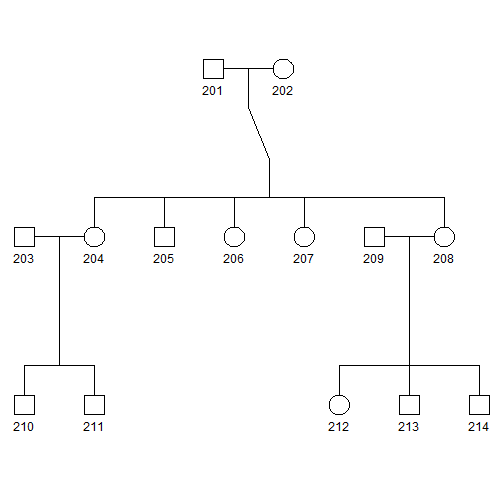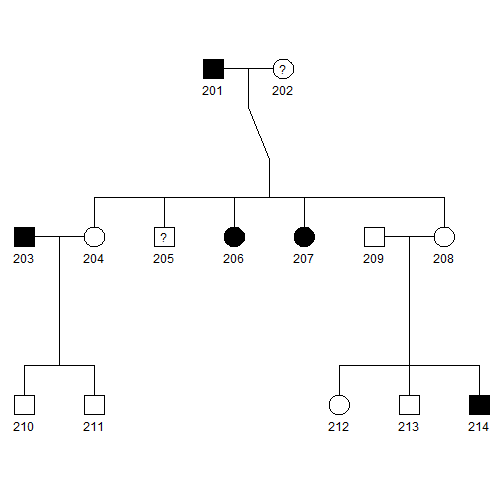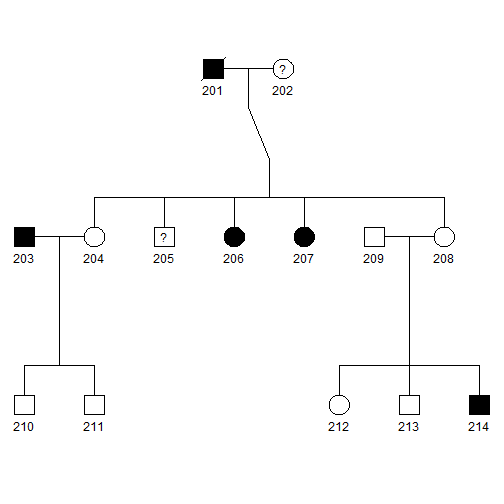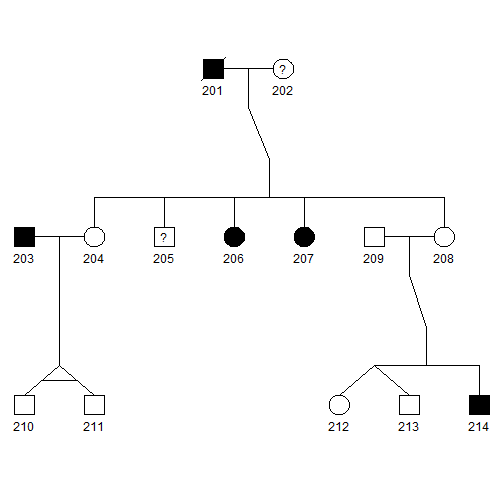# Pedigree plot using {kinship2}

| category RStudy  | tag Genetics
library(kinship2)
data(sample.ped)
sample.ped2 = subset(sample.ped, ped == 2)
sample.ped2

ped  id father mother sex affected avail
42   2 201      0      0   1        1     1
43   2 202      0      0   2       NA     0
44   2 203      0      0   1        1     1
45   2 204    201    202   2        0     1
46   2 205    201    202   1       NA     0
47   2 206    201    202   2        1     1
48   2 207    201    202   2        1     1
49   2 208    201    202   2        0     0
50   2 209      0      0   1        0     0
51   2 210    203    204   1        0     0
52   2 211    203    204   1        0     1
53   2 212    209    208   2        0     1
54   2 213    209    208   1        0     0
55   2 214    209    208   1        1     1


### Simple plot

pedAll <- pedigree(id = sample.ped2$id, dadid = sample.ped2$father, momid = sample.ped2$mother, sex = sample.ped2$sex, famid = sample.ped2$ped) print(pedAll)  Pedigree list with 14 total subjects in 1 families  print(pedAll["2"])  Pedigree object with 14 subjects, family id= 2 Bit size= 16  plot(pedAll["2"])Since there is only one family, or pedAll <- pedigree(id = sample.ped2$id, dadid = sample.ped2$father, momid = sample.ped2$mother,
sex = sample.ped2$sex, ) print(pedAll)  Pedigree object with 14 subjects Bit size= 16  plot(pedAll)• Squares and circles indicate males and females, respectively. ### Add affection status pedAll <- pedigree(id = sample.ped2$id, dadid = sample.ped2$father, momid = sample.ped2$mother,
sex = sample.ped2$sex, affected = sample.ped2$affected)
print(pedAll)

Pedigree object with 14 subjects
Bit size= 16

plot(pedAll)• Blackened squares and circles indicate the affected males and females, respectively.
• Squares and circles with a central question mark represent affection status is unknown.

Suppose the first individual (ID=201) is dead.

sample.ped2$status = c(1, rep(0, 13)) pedAll <- pedigree(id = sample.ped2$id, dadid = sample.ped2$father, momid = sample.ped2$mother,
sex = sample.ped2$sex, affected = sample.ped2$affected, status = sample.ped2$status) print(pedAll)  Pedigree object with 14 subjects Bit size= 16  plot(pedAll)• Squares and circles with a slash represent deceased. ### Add relationship (1=Monozygotic twin, 2=Dizygotic twin, 3=Twin of unknown zygosity, 4=Spouse) Suppose 210 and 211 are monozygotic twin, 212 and 213 are dizygotic twin, create a matrix with 3 columns (id1, id2, code) specifying special relationship between pairs of individuals. id1 = c(210, 212) id2 = c(211, 213) r = c(1, 2) rm = cbind(id1, id2, r)  pedAll <- pedigree(id = sample.ped2$id, dadid = sample.ped2$father, momid = sample.ped2$mother,
sex = sample.ped2$sex, affected = sample.ped2$affected, status = sample.ped2\$status,
relation = rm)
print(pedAll)

Pedigree object with 14 subjects
Bit size= 16

plot(pedAll)